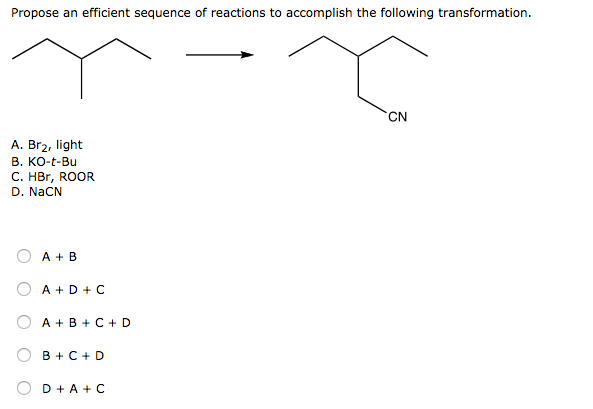# Problem: Propose an efficient sequence of reactions to accomplish the following transformation. a. A + B b. A + D + C c. A + B + C + D d. B + C + D e. D + A + C

###### FREE Expert Solution
87% (62 ratings)###### Problem Details

Propose an efficient sequence of reactions to accomplish the following transformation.

a. A + B

b. A + D + C

c. A + B + C + D

d. B + C + D

e. D + A + C